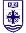###Summer Session Bulletin 2019

The grades and points of the University of Portland system are as follows:

 A = 4.0 B- = 2.7 D+ = 1.3 A- = 3.7 C+ = 2.3 D = 1.0 B+ = 3.3 C = 2.0 D- = .7 B = 3.0 C- = 1.7 F = 0